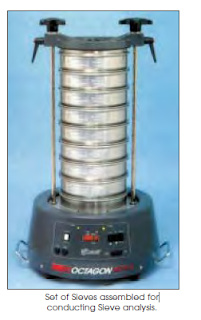## Pages

----------------------------------------------------------

## Saturday, 3 February 2018

### Grading of Aggregates

Aggregate comprises about 55 per cent of the volume of mortar and about 85 per cent
volume of mass concrete. Mortar contains aggregate of size of 4.75 mm and concrete contains aggregate upto a maximum size of 150 mm.

One of the practical methods of arriving at the practical grading by trial and error method is to mix aggregates of different size fractions in different percentages and to choose the one sample which gives maximum weight or minimum voids per unit volume, out of all the alternative samples. Fractions which are actually available in the field, or which could be made available in the field including that of the fine aggregate will be used in making samples.

Sieve Analysis
This is the name given to the operation of dividing a sample of aggregate into variousfractions each consisting of particles of the same size. The sieve analysis is conducted to determine the particle size distribution in a sample
of aggregate, which we call gradation.
A convenient system of expressing the
gradation of aggregate is one which the
consecutive sieve openings are constantly doubled,
such as 10 mm, 20 mm, 40 mm etc. Under such a
system, employing a logarithmic scale, lines can be
spaced at equal intervals to represent the successive
sizes.
The aggregates used for making concrete are
normally of the maximum size 80 mm, 40 mm, 20
mm, 10 mm, 4.75 mm, 2.36 mm, 600 micron, 300
micron and 150 micron. The aggregate fraction
from 80 mm to 4.75 mm are termed as coarse
aggregate and those fraction from 4.75 mm to 150
micron are termed as fine aggregate. The size 4.75
mm is a common fraction appearing both in coarse
aggregate and fine aggregate (C.A. and F.A.).
Mechanical devices are actually designed to
give motion in all possible direction, and as such, it is more systematic and efficient than handsieving.
For assessing the gradation by sieve analysis, the quantity of materials to be taken on
the sieve is given Table 3.8.
Many a time, fine aggregates are designated as coarse sand, medium sand and fine
sand. These classifications do not give any precise meaning. What the supplier terms as fine
sand may be really medium or even coarse sand. To avoid this ambiguity fineness modulus could be used as a yard stick to indicate the fineness of sand.
The following limits may be taken as guidance:
Fine sand : Fineness Modulus : 2.2 - 2.6
Medium sand : F.M. : 2.6 - 2.9
Coarse sand : F.M. : 2.9 - 3.2
A sand having a fineness modulus more than 3.2 will be unsuitable for making
satisfactory concrete.

Specific Surface and Surface Index

The importance of a good grading of the coarse and fine aggregate has already been
discussed. The quantity of water required to produce a given workability depends to a large extent on the surface area of the aggregate.
The total surface index (fx) of a mixture of aggregate is calculated by multiplying the

percentage of material retained on its sieve by the corresponding surface index and to their sum is added a constant of 330 and the result is divided by 1000. The method of computing the total surface for any given grading is shown in Table 3.13

Surface Index (fx) =

507/1000
= 0.507
Similarly, surface index can be calculated for standard grading curve, and this value of surface index can be taken as the desirable surface index of the combined aggregate. This parameter of surface index can be made use of for finding out the proportion of fine aggregate to coarse aggregate available in the field to obtain specified or desirable surface index in the following way.
Let x = surface index of fine aggregate
y = surface index of coarse aggregate
z = surface index of combined aggregate
a = proportion of fine to coarse aggregate
Then a = (z-y )/(x-z)
The following example will show how to combine the available fine aggregate with available coarse aggregate whose grading patterns are known to get the desirable surface index of the combined aggregate. The desirable surface index of the combined aggregate could be calculated from the grading pattern of the standard grading curve.